Get instant live expert help with Excel or Google Sheets“My Excelchat expert helped me in less than 20 minutes, saving me what would have been 5 hours of work!”

#### Post your problem and you’ll get expert help in seconds.

Your message must be at least 40 characters
Our professional experts are available now. Your privacy is guaranteed.

# Frequency distribution in Excel – Excelchat

A frequency distribution table and chart provide an easy way to present how our data is spread out over a specified range.  Through pivot tables, Excel allows us to create a frequency distribution table and chart.

Below is an example of a frequency distribution chart created through pivot tables.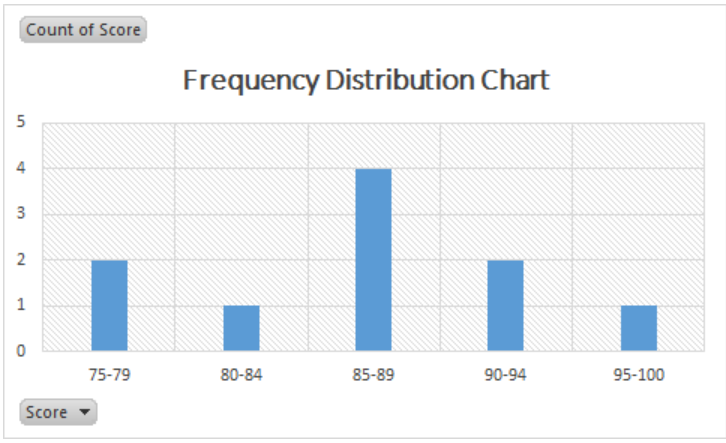Figure 1.  Final result: Frequency distribution

## How to create a frequency distribution

Here are the steps to make a frequency distribution:

• Prepare our data
• Insert a pivot table
• Insert a frequency distribution table
• Insert a frequency distribution chart

### Prepare our data

Below is a list of names and scores that we want to present as a frequency table and a frequency chart.Figure 2.  Sample data for frequency distribution

### Insert pivot table

We insert a pivot table by selecting our data (B2:C12), then clicking Insert tab > PivotTable.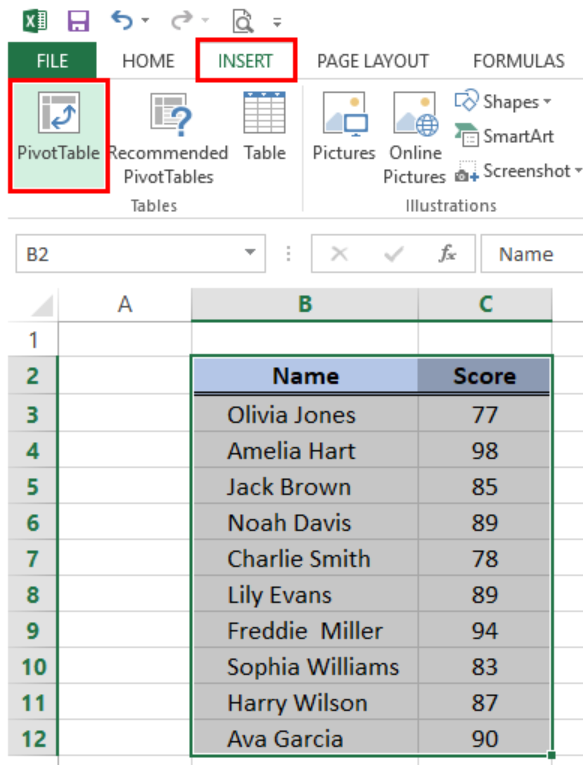Figure 3.  PivotTable option in Insert tab

In the Create PivotTable dialog box:

• Tick Existing Worksheet
• In Location, click the button and select cell E2

This will insert the pivot table in the existing worksheet in cell E2.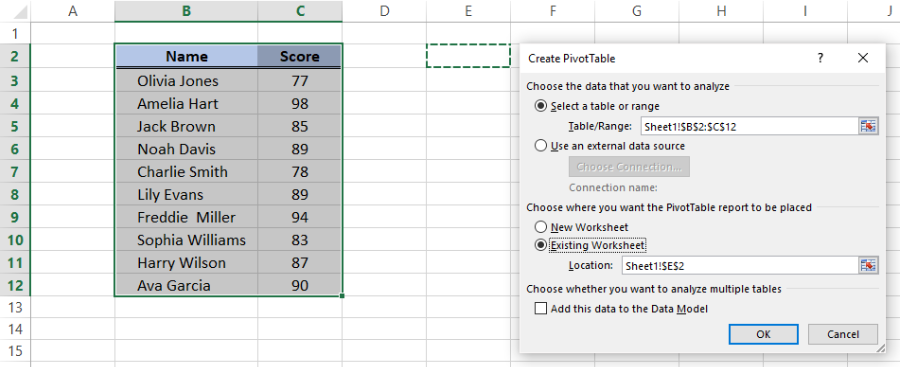Figure 4.  Create Pivot Table dialog box

• In order to create a pivot table that shows the scores, tick and drag the Score field to the box for ROWS and VALUES.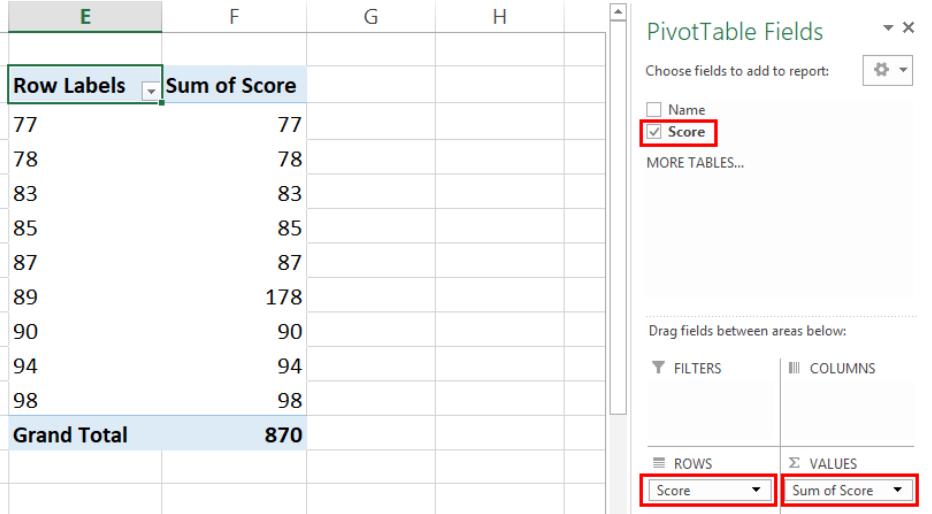Figure 5.  Drag and drop the Score field

• Under VALUES, let us change the function from SUM to COUNT by clicking the down arrow in Sum of Score and selecting Value Field Settings.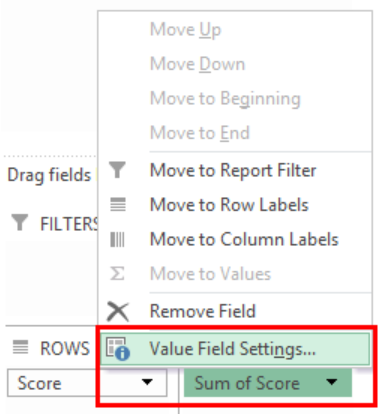Figure 6.  Value Field Settings option

• Choose from the type of calculation and select Count.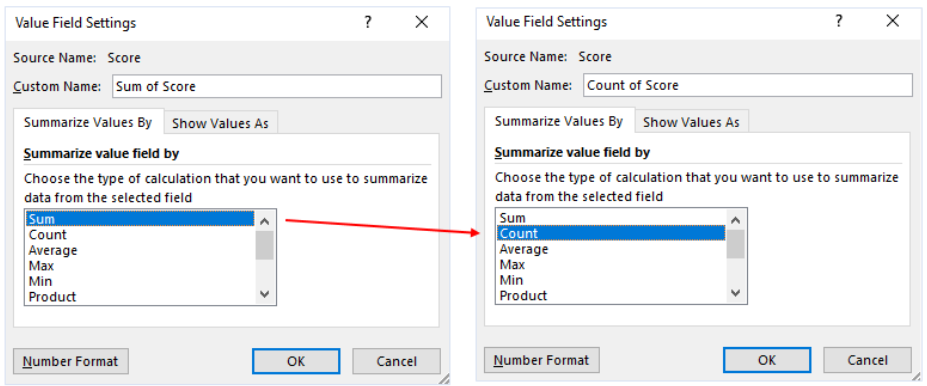Figure 7.  Change type of calculation from Sum to Count

We have now created a pivot table showing the count per score.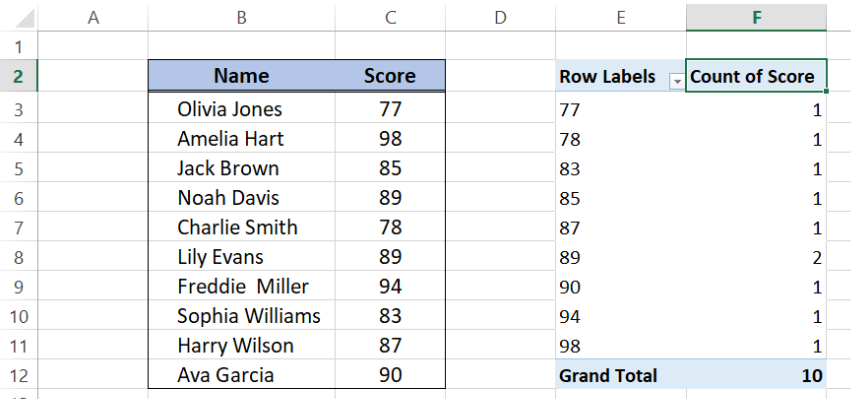Figure 8.  Pivot table created

## How to create a frequency distribution table

• Click anywhere under Row Labels and select Group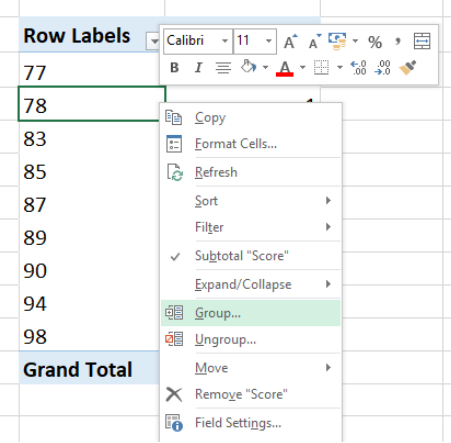Figure 9.  Select Group in menu options

The grouping default values will be the minimum and maximum values in our list, and grouping is set by 1.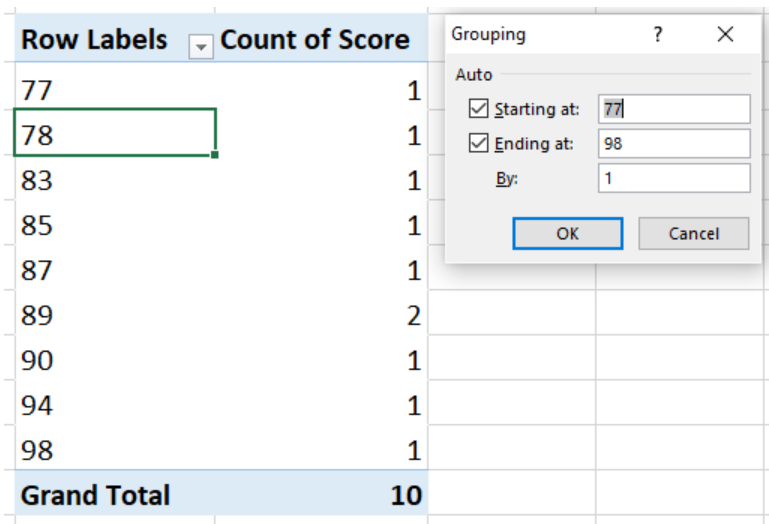Figure 10.  Default grouping parameters

For this example, we want to group the scores by 5, starting from 75 to 100.

Enter the following in the textboxes:

• Starting at: 75
• Ending at: 100
• By: 5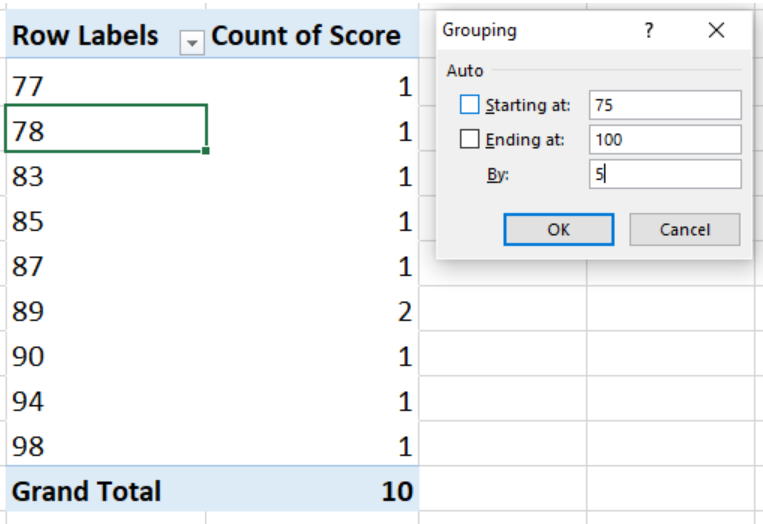Figure 11. Customize grouping parameters

Click OK and the frequency distribution table will be created, showing the frequency or count of scores per specific range.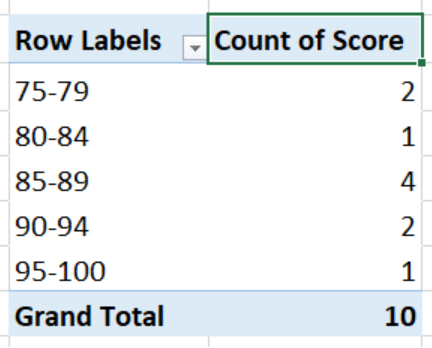Figure 12.  Output: How to create a frequency distribution table

## How to create a frequency distribution chart

Click anywhere on the pivot table then click Insert tab > PivotChartFigure 13.  PivotChart in Insert tab

The Insert Chart dialog box will appear.  Select the Clustered Column chart type and click OK.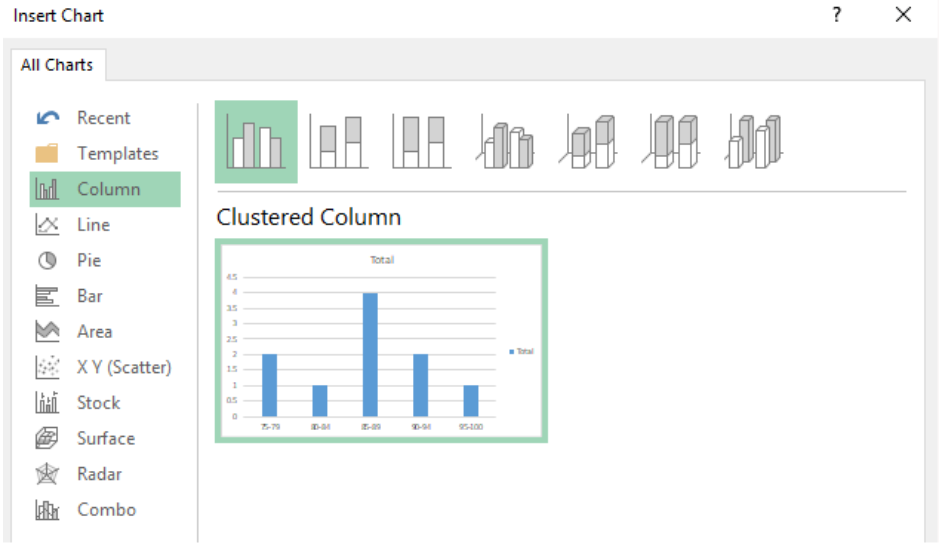Figure 14.  Insert Clustered Column chart

We will instantly create a frequency distribution chart, showing the score ranges in the horizontal axis and the corresponding frequencies in the vertical axis.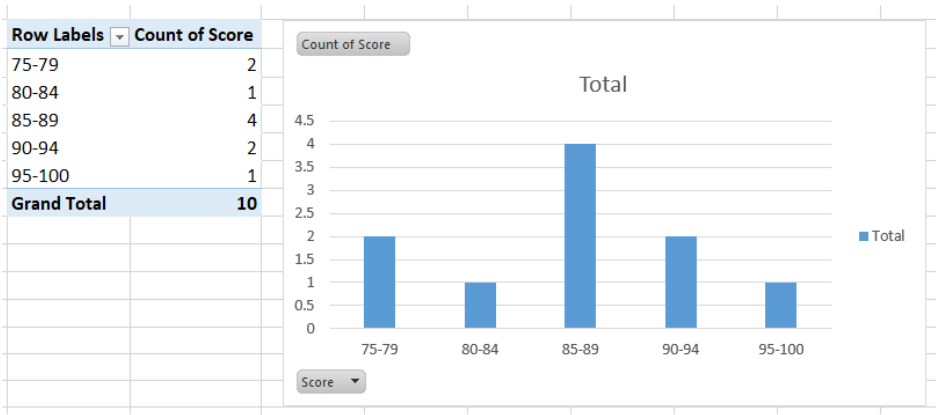Figure 15.  Output: Create frequency distribution chart

We can customize our frequency distribution chart by performing the following:

• Change chart title to Scores Frequency Distribution
• Delete the legend
• Format the vertical axis (set maximum value to 5.0, major units to 1.0)
• Change the chart design to Style 8

Finally, we have created a frequency distribution chart, as shown below.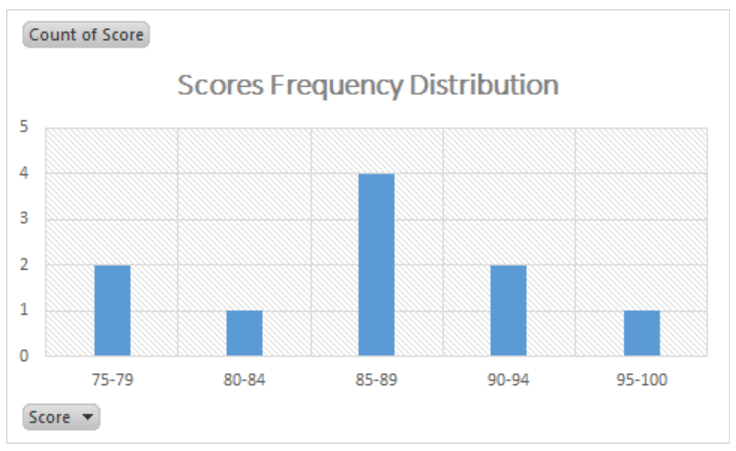Figure 16.  Output: Create frequency distribution

## Instant Connection to an Excel Expert

Most of the time, the problem you will need to solve will be more complex than a simple application of a formula or function. If you want to save hours of research and frustration, try our live Excelchat service! Our Excel Experts are available 24/7 to answer any Excel question you may have. We guarantee a connection within 30 seconds and a customized solution within 20 minutes.